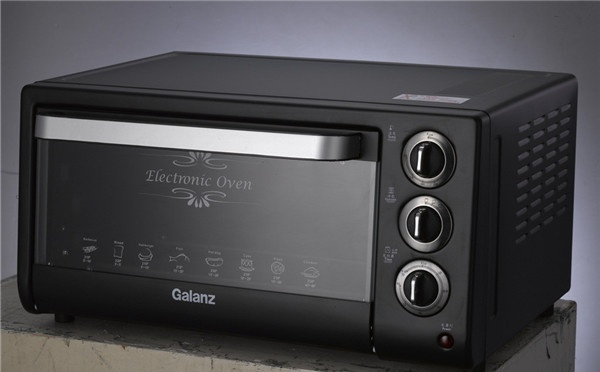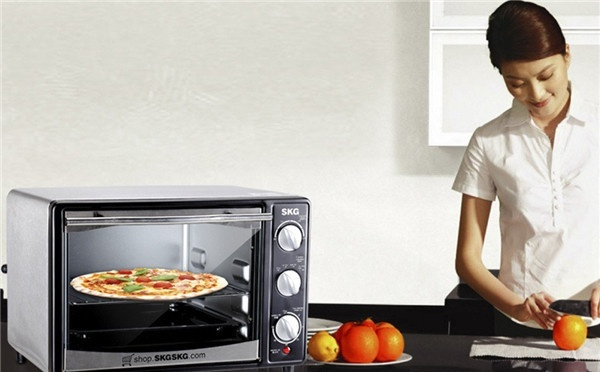|

# 电烤箱怎么用 电烤箱正确的使用方法

现今各种小家电不断的被推出，电烤箱的种类和功能也越发的丰富。基本上每家每户都有一台电烤箱，平时你们都会用电烤箱做一些什么食物呢？了解电烤箱怎么用吗？

【电烤箱怎么用】

电烤箱的烤盘和烤网主要用于烘烤食物的时候使用，在烤制任何食物的时候，一次只能放置一个烤盘或者烤网，有助于食物同时受到上下火的烘烤。在使用电烤箱烤制食物的时候先将调制好待烘烤的食品放人已用食油涂擦过的烤盘内，再插上电源插头，将转换开关拧至满负荷档，即上下加热同时通电，并将调温器拧至所需的温度位置。经过一定时间，指示灯熄灭，表示烤箱已达到预热温度。当供烤到达预定时间内，将转换开关、调温器转回“关”位置，拔去电源插头，取出食品，待烤箱冷却后，将炉腔，沪门和附件擦抹千净。家用电烤箱的正确使用，关系到家人的安全。所以在使用电烤箱的时候，一定要掌握电烤箱怎么用。在首次用电烤箱的时候一定要详细的阅读电烤箱的使用说明后才使用，初次使用电烤箱是要把电烤箱里内外进行一次全方位的消毒和清洁。在刚开始使用电烤箱的时候，会有一点异味，这是正常现象，使用几次以后就没有了。

【家庭电烤箱的用途】

1、它能制作出我们最喜欢的烤肉或者是烤鸭等，只要我们将买回来的食材制作好，放进电烤箱内，选择好相应的按钮，制定好烤制时间，只要等时间一到，就能享受到美味的烤肉了，还没有油烟，整个厨房显得干净清爽。

2、小孩子最喜欢的就是零食了，很多的大人也不例外，电烤箱还能够烤制披萨、饼干和面包。如果早上起床的比较晚了，就可以用电烤箱烤制面包。用电烤箱烤制面包和饼干等零食等待的时间短，还非常的美味。3、除此之外，我们平时买回来的瓜子、饼干等食物在家放置一段时间就会受潮，影响食物的口感，同时这些食物的保存时间也会变短，而电烤箱就能完美的解决这些问题，它能将受潮的食物经过加热，将食物里面的水分烘干，重新制成我们刚买的时候的状态，还能保证食物的味道不散失。

4、电烤箱还有一个用途是发酵，我们将需要发酵的面团放入电烤箱之后把温度调低，食物在电烤箱里会很快的发酵。利用这个原理，我们还可以使用电烤箱制作巧克力。

【电烤箱的正确操作步骤】

（1）将调制好待烘烤的食品放人已用食油涂擦过的烤盘内；

（2）插上电源插头；

（3）将转换开关拧至满负荷档，即上下加热同时通电，并将调温器拧至所需的温度位置。经过一定时间，指示灯熄灭，表示烤箱已达到预热温度。（4）用柄叉将已放有待供烤食品的烤盘放进烤箱内。

（5）需要自动定时控制时，将定时器拧至预定的烘烤时间；若不需要自动定时控制，则将定时器拧至“长接”的位置。

（6）烘烤过程中应随时观察食品各部分受热是否均匀，必要时用柄叉将烤盘调转方向。

（7）当供烤到达预定时间内，将转换开关、调温器转回“关”位置，拔去电源插头，取出食品，待烤箱冷却后，将炉腔，沪门和附件擦抹千净。

【电烤箱使用中的保养方法】

1、在使用电烤箱之前，应仔细阅读使用说明书，并核查一下当地使用电源电压是否与电烤箱的要求相一致。

2、将要放入烤箱内烘烤的食品预先用作料调配好，并按需要盛装妥当。烘烤排骨、鸡、鸭、肉类、面包、馅饼等，应 将其放入烤盘内；烤鱼时要先将鱼放在烤网上再放入烤盘。烤盘使用前最好涂一些食油或铺上一层铝箔纸，以便于取出食物和用后清洁。

3、烤盘放在烤箱内腔的位置高低，可根据食品的大小和烤制方法来选择。一般来说，上层的温度稍高，中层适中，下层较低。

4、首先把自动温度调节器旋钮、定时器旋钮、转换开关等调至最低位置，接通电源，指示灯亮。待箱内加热后，再按箜烤食物所要求的温度、时间和功率调节相应的旋钮至需要的位置。5、当指示灯熄灭，箱内已达到预热温度时，打开箱门，持柄叉盛放食物的烤盘网放入烤箱内适当位置，并关好箱门，开启烘烤开关。

6、通过窗口观察烘烤食物的各部分是否受热均匀，必要时，可用柄叉将烤盘调转方向。体积大，肉层厚的食物，在烘烤过程中最好适当翻动1——2次，这样，会烤的更均匀些。

7、取放烤箱的食物时，一定要用柄叉连同烤盘或烤网一起取放，禁止用手直接 操作，以免烫伤。

8、使用完毕，应及时拔下电源插头。

`声明：本文由入驻焦点开放平台的作者撰写，除焦点官方账号外，观点仅代表作者本人，不代表焦点立场错误信息举报电话： 400-099-0099，邮箱：jubao@vip.sohu.com，或点此进行意见反馈，或点此进行举报投诉。`A B C D E F G H J K L M N P Q R S T W X Y Z
A - B - C - D - E
• A
• 鞍山
• 安庆
• 安阳
• 安顺
• 安康
• 澳门
• B
• 北京
• 保定
• 包头
• 巴彦淖尔
• 本溪
• 蚌埠
• 亳州
• 滨州
• 北海
• 百色
• 巴中
• 毕节
• 保山
• 宝鸡
• 白银
• 巴州
• C
• 承德
• 沧州
• 长治
• 赤峰
• 朝阳
• 长春
• 常州
• 滁州
• 池州
• 长沙
• 常德
• 郴州
• 潮州
• 崇左
• 重庆
• 成都
• 楚雄
• 昌都
• 慈溪
• 常熟
• D
• 大同
• 大连
• 丹东
• 大庆
• 东营
• 德州
• 东莞
• 德阳
• 达州
• 大理
• 德宏
• 定西
• 儋州
• 东平
• E
• 鄂尔多斯
• 鄂州
• 恩施
F - G - H - I - J
• F
• 抚顺
• 阜新
• 阜阳
• 福州
• 抚州
• 佛山
• 防城港
• G
• 赣州
• 广州
• 桂林
• 贵港
• 广元
• 广安
• 贵阳
• 固原
• H
• 邯郸
• 衡水
• 呼和浩特
• 呼伦贝尔
• 葫芦岛
• 哈尔滨
• 黑河
• 淮安
• 杭州
• 湖州
• 合肥
• 淮南
• 淮北
• 黄山
• 菏泽
• 鹤壁
• 黄石
• 黄冈
• 衡阳
• 怀化
• 惠州
• 河源
• 贺州
• 河池
• 海口
• 红河
• 汉中
• 海东
• 怀来
• I
• J
• 晋中
• 锦州
• 吉林
• 鸡西
• 佳木斯
• 嘉兴
• 金华
• 景德镇
• 九江
• 吉安
• 济南
• 济宁
• 焦作
• 荆门
• 荆州
• 江门
• 揭阳
• 金昌
• 酒泉
• 嘉峪关
K - L - M - N - P
• K
• 开封
• 昆明
• 昆山
• L
• 廊坊
• 临汾
• 辽阳
• 连云港
• 丽水
• 六安
• 龙岩
• 莱芜
• 临沂
• 聊城
• 洛阳
• 漯河
• 娄底
• 柳州
• 来宾
• 泸州
• 乐山
• 六盘水
• 丽江
• 临沧
• 拉萨
• 林芝
• 兰州
• 陇南
• M
• 牡丹江
• 马鞍山
• 茂名
• 梅州
• 绵阳
• 眉山
• N
• 南京
• 南通
• 宁波
• 南平
• 宁德
• 南昌
• 南阳
• 南宁
• 内江
• 南充
• P
• 盘锦
• 莆田
• 平顶山
• 濮阳
• 攀枝花
• 普洱
• 平凉
Q - R - S - T - W
• Q
• 秦皇岛
• 齐齐哈尔
• 衢州
• 泉州
• 青岛
• 清远
• 钦州
• 黔南
• 曲靖
• 庆阳
• R
• 日照
• 日喀则
• S
• 石家庄
• 沈阳
• 双鸭山
• 绥化
• 上海
• 苏州
• 宿迁
• 绍兴
• 宿州
• 三明
• 上饶
• 三门峡
• 商丘
• 十堰
• 随州
• 邵阳
• 韶关
• 深圳
• 汕头
• 汕尾
• 三亚
• 三沙
• 遂宁
• 山南
• 商洛
• 石嘴山
• T
• 天津
• 唐山
• 太原
• 通辽
• 铁岭
• 泰州
• 台州
• 铜陵
• 泰安
• 铜仁
• 铜川
• 天水
• 天门
• W
• 乌海
• 乌兰察布
• 无锡
• 温州
• 芜湖
• 潍坊
• 威海
• 武汉
• 梧州
• 渭南
• 武威
• 吴忠
• 乌鲁木齐
X - Y - Z
• X
• 邢台
• 徐州
• 宣城
• 厦门
• 新乡
• 许昌
• 信阳
• 襄阳
• 孝感
• 咸宁
• 湘潭
• 湘西
• 西双版纳
• 西安
• 咸阳
• 西宁
• 仙桃
• 西昌
• Y
• 运城
• 营口
• 盐城
• 扬州
• 鹰潭
• 宜春
• 烟台
• 宜昌
• 岳阳
• 益阳
• 永州
• 阳江
• 云浮
• 玉林
• 宜宾
• 雅安
• 玉溪
• 延安
• 榆林
• 银川
• Z
• 张家口
• 镇江
• 舟山
• 漳州
• 淄博
• 枣庄
• 郑州
• 周口
• 驻马店
• 株洲
• 张家界
• 珠海
• 湛江
• 肇庆
• 中山
• 自贡
• 资阳
• 遵义
• 昭通
• 张掖
• 中卫

1室1厅1厨1卫1阳台

1
2
3
4
5

0
1
2

1

1

0
1
2
3报名成功，资料已提交审核A B C D E F G H J K L M N P Q R S T W X Y Z
A - B - C - D - E
• A
• 鞍山
• 安庆
• 安阳
• 安顺
• 安康
• 澳门
• B
• 北京
• 保定
• 包头
• 巴彦淖尔
• 本溪
• 蚌埠
• 亳州
• 滨州
• 北海
• 百色
• 巴中
• 毕节
• 保山
• 宝鸡
• 白银
• 巴州
• C
• 承德
• 沧州
• 长治
• 赤峰
• 朝阳
• 长春
• 常州
• 滁州
• 池州
• 长沙
• 常德
• 郴州
• 潮州
• 崇左
• 重庆
• 成都
• 楚雄
• 昌都
• 慈溪
• 常熟
• D
• 大同
• 大连
• 丹东
• 大庆
• 东营
• 德州
• 东莞
• 德阳
• 达州
• 大理
• 德宏
• 定西
• 儋州
• 东平
• E
• 鄂尔多斯
• 鄂州
• 恩施
F - G - H - I - J
• F
• 抚顺
• 阜新
• 阜阳
• 福州
• 抚州
• 佛山
• 防城港
• G
• 赣州
• 广州
• 桂林
• 贵港
• 广元
• 广安
• 贵阳
• 固原
• H
• 邯郸
• 衡水
• 呼和浩特
• 呼伦贝尔
• 葫芦岛
• 哈尔滨
• 黑河
• 淮安
• 杭州
• 湖州
• 合肥
• 淮南
• 淮北
• 黄山
• 菏泽
• 鹤壁
• 黄石
• 黄冈
• 衡阳
• 怀化
• 惠州
• 河源
• 贺州
• 河池
• 海口
• 红河
• 汉中
• 海东
• 怀来
• I
• J
• 晋中
• 锦州
• 吉林
• 鸡西
• 佳木斯
• 嘉兴
• 金华
• 景德镇
• 九江
• 吉安
• 济南
• 济宁
• 焦作
• 荆门
• 荆州
• 江门
• 揭阳
• 金昌
• 酒泉
• 嘉峪关
K - L - M - N - P
• K
• 开封
• 昆明
• 昆山
• L
• 廊坊
• 临汾
• 辽阳
• 连云港
• 丽水
• 六安
• 龙岩
• 莱芜
• 临沂
• 聊城
• 洛阳
• 漯河
• 娄底
• 柳州
• 来宾
• 泸州
• 乐山
• 六盘水
• 丽江
• 临沧
• 拉萨
• 林芝
• 兰州
• 陇南
• M
• 牡丹江
• 马鞍山
• 茂名
• 梅州
• 绵阳
• 眉山
• N
• 南京
• 南通
• 宁波
• 南平
• 宁德
• 南昌
• 南阳
• 南宁
• 内江
• 南充
• P
• 盘锦
• 莆田
• 平顶山
• 濮阳
• 攀枝花
• 普洱
• 平凉
Q - R - S - T - W
• Q
• 秦皇岛
• 齐齐哈尔
• 衢州
• 泉州
• 青岛
• 清远
• 钦州
• 黔南
• 曲靖
• 庆阳
• R
• 日照
• 日喀则
• S
• 石家庄
• 沈阳
• 双鸭山
• 绥化
• 上海
• 苏州
• 宿迁
• 绍兴
• 宿州
• 三明
• 上饶
• 三门峡
• 商丘
• 十堰
• 随州
• 邵阳
• 韶关
• 深圳
• 汕头
• 汕尾
• 三亚
• 三沙
• 遂宁
• 山南
• 商洛
• 石嘴山
• T
• 天津
• 唐山
• 太原
• 通辽
• 铁岭
• 泰州
• 台州
• 铜陵
• 泰安
• 铜仁
• 铜川
• 天水
• 天门
• W
• 乌海
• 乌兰察布
• 无锡
• 温州
• 芜湖
• 潍坊
• 威海
• 武汉
• 梧州
• 渭南
• 武威
• 吴忠
• 乌鲁木齐
X - Y - Z
• X
• 邢台
• 徐州
• 宣城
• 厦门
• 新乡
• 许昌
• 信阳
• 襄阳
• 孝感
• 咸宁
• 湘潭
• 湘西
• 西双版纳
• 西安
• 咸阳
• 西宁
• 仙桃
• 西昌
• Y
• 运城
• 营口
• 盐城
• 扬州
• 鹰潭
• 宜春
• 烟台
• 宜昌
• 岳阳
• 益阳
• 永州
• 阳江
• 云浮
• 玉林
• 宜宾
• 雅安
• 玉溪
• 延安
• 榆林
• 银川
• Z
• 张家口
• 镇江
• 舟山
• 漳州
• 淄博
• 枣庄
• 郑州
• 周口
• 驻马店
• 株洲
• 张家界
• 珠海
• 湛江
• 肇庆
• 中山
• 自贡
• 资阳
• 遵义
• 昭通
• 张掖
• 中卫• 手机• 分享
• 设计
免费设计
• 计算器
装修计算器
• 入驻
合作入驻
• 联系
联系我们
• 置顶
返回顶部